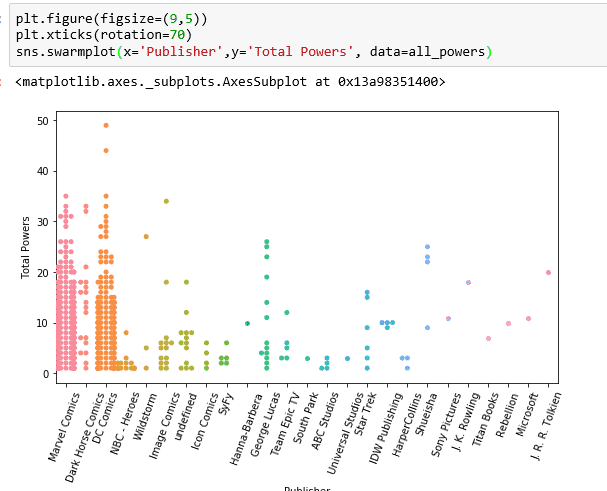# Which Superhero Has the Most Powers? | Data Viz

### The datasets

This data set was sourced from Kaggle. There are two data sets that had to be preprocessed and merged. The first data set contained each out the hero’s dimensions such as race, eye color, and other physical dimensions. The second dataset contains all the powers that are valued in boolean format.  The booleans were changed to zeros and ones in order to calculate the total powers.  Also, the powers had to be summed in a single column so that we could view all the powers.

### Check out the Power Bi Visual:

Check Out: Marvio Vs Zelda Sales Data Viz

```#import dependencies
import pandas as pd
import numpy as np
import matplotlib.pyplot as plt
import seaborn as sns
%matplotlib inline

# load in the data sets

### What are the alignments have the most heroes by gender?

Now that the data is loaded, let’s start to visualize some of the data. I would like to see what the proportion of good and evil alignment based on gender using a seaborn catplot and a violinplot.

`sns.catplot(x='hero_type',y='Total Powers',hue='Gender',data=all_powers)``sns.violinplot(x='hero_type',y='Total Powers',data=all_powers)`### Which publisher’s heroes have the most powers?

```plt.figure(figsize=(9,5))
plt.xticks(rotation=70)
sns.swarmplot(x='Publisher',y='Total Powers', data=all_powers)```## Watch the Video on Python Data Visualization

• in
• |
• May 27, 2019#### Gaelim Holland

Subscribe
Notify of